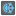• # Want some ideas

## Question related to missionColder-Warmer

Hello everyone,

I cannot pass the test 2 cause I exceed the limited step length. I have no idea at this moment.

Could you help review my code and give me your ideas?

```from math import hypot
MAX_STEP = 12
def check_solution(func, goal, start):
prev_steps = [start]
for step in range(MAX_STEP):
row, col = func([s[:] for s in prev_steps])
if [row, col] == goal:
return True
if 10 <= row or 0 > row or 10 <= col or 0 > col:
print("You gave wrong coordinates.")
return False
prev_distance = hypot(prev_steps[-1] - goal, prev_steps[-1] - goal)
distance = hypot(row - goal, col - goal)
alteration = 0 if prev_distance == distance else (1 if prev_distance > distance else -1)
prev_steps.append([row, col, alteration])
print("Too many steps")
return False

from itertools import product
def checkio(steps):
x, y, status = steps[-1]
log = {(x, y): warmer for x, y, warmer in steps}
print(steps, log)
for dx, dy in product((1, -1, 0), repeat = 2):
if 0 <= x + dx < 10 and 0 <= y + dy < 10:
if status == -1:
x, y = [(px, py) for px, py, warmer in steps if warmer != -1][-1]
nextStep = (x + dx, y + dy)
if nextStep not in log.keys():
return nextStep

print(check_solution(checkio, [5, 6], [0, 0, 0]))    # test 2
```30